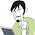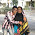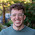### Object tracking in Java - detect position of colored spot in imageRed spot in image - position to be detected later
Object Tracking plays important role in Image Processing research projects. In this example, I am showing how we can detect the position [(x, y) coordinates ] of a colored spot in given image using JavaCV (Java wrapper for OpenCV ).

Input image :
This image has a red colored spot. And our objective is to track the position coordinate of the spot in image.The example below uses thresholding in HSV space and simple moment calculations given in OpenCV library.

You can use this code to track an object in a video sequence - say live web-cam capture video.

Detecting Position of a spot in Threshold image:
``````    static Dimension getCoordinates(IplImage thresholdImage) {
int posX = 0;
int posY = 0;        CvMoments moments = new CvMoments();
cvMoments(thresholdImage, moments, 1);
// cv Spatial moment : Mji=sumx,y(I(x,y)•xj•yi)
// where I(x,y) is the intensity of the pixel (x, y).
double momX10 = cvGetSpatialMoment(moments, 1, 0); // (x,y)
double momY01 = cvGetSpatialMoment(moments, 0, 1);// (x,y)
double area = cvGetCentralMoment(moments, 0, 0);
posX = (int) (momX10 / area);
posY = (int) (momY01 / area);
return new Dimension(posX, posY);
}
``````
The complete working source  Code:
``````//imports
import static com.googlecode.javacv.cpp.opencv_core.cvCreateImage;
import static com.googlecode.javacv.cpp.opencv_core.cvGetSize;
import static com.googlecode.javacv.cpp.opencv_core.cvInRangeS;
import static com.googlecode.javacv.cpp.opencv_core.cvReleaseImage;
import static com.googlecode.javacv.cpp.opencv_core.cvScalar;
import static com.googlecode.javacv.cpp.opencv_highgui.cvLoadImage;
import static com.googlecode.javacv.cpp.opencv_highgui.cvSaveImage;
import static com.googlecode.javacv.cpp.opencv_imgproc.CV_BGR2HSV;
import static com.googlecode.javacv.cpp.opencv_imgproc.CV_MEDIAN;
import static com.googlecode.javacv.cpp.opencv_imgproc.cvCvtColor;
import static com.googlecode.javacv.cpp.opencv_imgproc.cvSmooth;
import static com.googlecode.javacv.cpp.opencv_imgproc.cvGetSpatialMoment;
import static com.googlecode.javacv.cpp.opencv_imgproc.cvGetCentralMoment;
import static com.googlecode.javacv.cpp.opencv_imgproc.cvMoments;
import java.awt.Dimension;

import com.googlecode.javacv.cpp.opencv_core.IplImage;
import com.googlecode.javacv.cpp.opencv_imgproc.CvMoments;

public class ObjectPositionDetect {
static int hueLowerR = 160;
static int hueUpperR = 180;

public static void main(String[] args) {
IplImage orgImg = cvLoadImage("finding-red-color-spot.JPG");
IplImage thresholdImage = hsvThreshold(orgImg);
cvSaveImage("hsvthreshold.jpg", thresholdImage);
Dimension position = getCoordinates(thresholdImage);
System.out.println("Dimension of original Image : " + thresholdImage.width() + " , " + thresholdImage.height());
System.out.println("Position of red spot    : x : " + position.width + " , y : " + position.height);
}

static Dimension getCoordinates(IplImage thresholdImage) {
int posX = 0;
int posY = 0;
CvMoments moments = new CvMoments();
cvMoments(thresholdImage, moments, 1);
// cv Spatial moment : Mji=sumx,y(I(x,y)•xj•yi)
// where I(x,y) is the intensity of the pixel (x, y).
double momX10 = cvGetSpatialMoment(moments, 1, 0); // (x,y)
double momY01 = cvGetSpatialMoment(moments, 0, 1);// (x,y)
double area = cvGetCentralMoment(moments, 0, 0);
posX = (int) (momX10 / area);
posY = (int) (momY01 / area);
return new Dimension(posX, posY);
}

static IplImage hsvThreshold(IplImage orgImg) {
// 8-bit, 3- color =(RGB)
IplImage imgHSV = cvCreateImage(cvGetSize(orgImg), 8, 3);
System.out.println(cvGetSize(orgImg));
cvCvtColor(orgImg, imgHSV, CV_BGR2HSV);
// 8-bit 1- color = monochrome
IplImage imgThreshold = cvCreateImage(cvGetSize(orgImg), 8, 1);
// cvScalar : ( H , S , V, A)
cvInRangeS(imgHSV, cvScalar(hueLowerR, 100, 100, 0), cvScalar(hueUpperR, 255, 255, 0), imgThreshold);
cvReleaseImage(imgHSV);
cvSmooth(imgThreshold, imgThreshold, CV_MEDIAN, 13);
// save
return imgThreshold;
}
}

``````
Outputs :
Thresolding in HSV space : also given in my earlier postOutput of image thresholding in hsv - of image above
Detected Position :

Dimension of original Image : 800 , 600
Position of red spot : x : 84 , y : 320
Demo Video: Drawing by tracking the position of red object

#### 13 comments :

1.I am studying at Pulchowk campus (BCT). JavaCV seems to be very interesting but it does not come with a documentation. How did you learn it? I read the code of many samples but I did not understand most of the lines.

1.Robik, I am glad that you are also from Pulchowk campus.

Yes, JavaCV has fewer resources on the web.
But there is also active community like google group : https://groups.google.com/group/javacv . You can join this group and participate/search/ask for help/samples/discussions.

The openCV's documentation (http://opencv.willowgarage.com/documentation/cpp/index.html) helps a lot to understand JavaCV.

2.Thanks for the links.

2.Hi Ganesh,

Thanks a lot for your post.
Can you please point to a resource you used to get HSV values harcoded in your program?

thanks a lot in advance,
genia

3.Hi, I love this post. Exactly what I needed, even tho shape isn't that important.

But my question is, is this fast or do it take allot computer capacity? Would it be faster if i just search the color and return the position of the first spot of that color?

Thanks.

1.Hi Nille, I can't exactly say which one will be faster.
It may depend on how you do the search for the color.

2.Basically, your approach might not require all the operation like thresholding and moment calculations...
Please try it and let us know your result.

4.How to do to find one more point in image?

5.How can you detect the positions if there are more than one color to be detected. pls help.

6.Why it can not find the color in the dark? In HSV it should be red too, but it isn`t detected. Where i can change this to get it to work?

7.Thank you for sharing this project. I was wondering about one thing though. There are not many documentations or tutorials available in the net. How did you learn JavaCV?

8.I've put together the code for this tutorial, including a video feed. All the libraries are included, so the setup is very quick.
https://github.com/theicfire/OpenCVHueDetect

9.Great !
Thanks!!!

Your Comment and Question will help to make this blog better...